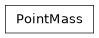# geoana.gravity.PointMass#class geoana.gravity.PointMass(mass=1.0, location=None, **kwargs)#

Class for gravitational solutions for a point mass.

The `PointMass` class is used to analytically compute the gravitational potentials, fields, and gradients for a point mass.

Parameters
massfloat

Mass of the point particle (kg). Default is m = 1 kg

locationarray_like, optional

Location of the point mass in 3D space (m). Default is (0, 0, 0)

Attributes

 `location` Location of the point mass. `mass` Mass of the point particle in kilograms.

Methods

 Gravitational field due to a point mass. Gravitational gradient for a point mass. Gravitational potential due to a point mass.